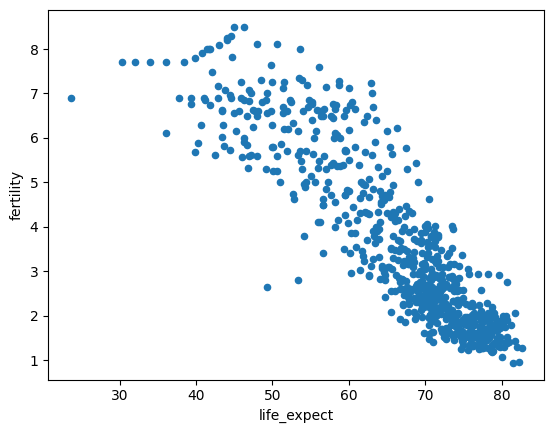# Plots¶

## `Plot`¶

Datapane supports all major Python visualization libraries, allowing you to add interactive plots and visualizations to your app.

The `dp.Plot` block takes a plot object from one of the supported Python visualization libraries and renders it in your app.

Info

Datapane will automatically wrap your visualization or plot in a `dp.Plot` block if you pass it into your app directly.

Parameters:

Name Type Description Default
`data` `Any`

The `plot` object to attach

required
`caption` `Optional[str]`

A caption to display below the plot (optional)

`None`
`responsive` `bool`

Whether the plot should automatically be resized to fit, set to False if your plot looks odd (optional, default: True)

`True`
`scale` `float`

Set the scaling factor for the plt (optional, default = 1.0)

`1.0`
`name` `BlockId`

A unique name for the block to reference when adding text or embedding (optional)

`None`
`label` `str`

A label used when displaying the block (optional)

`None`

## ¶

Datapane currently supports the following libraries:

If you're using another visualization library e.g. Pyvis for networks, try saving your chart as a local HTML file and wrapping that in a dp.HTML block.

## Altair¶

Altair is a declarative statistical visualization library for Python, based on Vega and Vega-Lite. Altair’s API is simple, friendly and consistent and built on top of the powerful Vega-Lite visualization grammar. This elegant simplicity produces beautiful and effective visualizations with a minimal amount of code.

To get started using Altair to make your visualizations, begin with Altair's Documentation

``````import altair as alt
import pandas as pd
from vega_datasets import data as vega_data

select_year = alt.selection_point(
name="select",
fields=["year"],
value={"year": 1955},
bind=alt.binding_range(min=1955, max=2005, step=5),
)

alt_chart = (
alt.Chart(gap)
.mark_point(filled=True)
.encode(
alt.X("fertility", scale=alt.Scale(zero=False)),
alt.Y("life_expect", scale=alt.Scale(zero=False)),
alt.Size("pop:Q"),
alt.Color("cluster:N"),
alt.Order("pop:Q", sort="descending"),
)
.transform_filter(select_year)
)

dp.Plot(alt_chart)
``````

## Bokeh¶

Bokeh is an interactive visualization library which provides elegant, concise construction of versatile graphics, and affords high-performance interactivity over large datasets.

To get started using Bokeh to make your visualizations, begin with Bokeh's User Guide.

``````from bokeh.plotting import figure, output_file, show
from bokeh.sampledata.iris import flowers

colormap = {"setosa": "red", "versicolor": "green", "virginica": "blue"}
colors = [colormap[x] for x in flowers["species"]]

bokeh_chart = figure(title="Iris Morphology")
bokeh_chart.xaxis.axis_label = "Petal Length"
bokeh_chart.yaxis.axis_label = "Petal Width"

bokeh_chart.circle(
flowers["petal_length"],
flowers["petal_width"],
color=colors,
fill_alpha=0.2,
size=10,
)

dp.Plot(bokeh_chart)
``````

## Matplotlib¶

Matplotlib is the original Python visualization library, often supported and used with Jupyter Notebooks. Matplotlib plots are not interactive in Datapane apps, but are saved as SVGs so can be viewed at high fidelity.

Higher-level matplotlib libraries such as Seaborn are also supported, and can be used in a similar way to the matplotlib example below,

``````import matplotlib.pyplot as plt
import pandas as pd
from vega_datasets import data as vega_data

fig = gap.plot.scatter(x="life_expect", y="fertility")

dp.Plot(fig)
``````Info

You can pass either a `matplotlib` `Figure` or `Axes` object to `dp.Plot`, you can obtain the current global figure from `matplotlib` by running `plt.gcf()`

## Plotly¶

Plotly's Python graphing library makes interactive, publication-quality graphs.

``````import plotly.express as px

df = px.data.gapminder()

plotly_chart = px.scatter(
df.query("year==2007"),
x="gdpPercap",
y="lifeExp",
size="pop",
color="continent",
hover_name="country",
log_x=True,
size_max=60,
)

dp.Plot(plotly_chart)
``````

## Folium¶

Folium makes it easy to visualize data that’s been manipulated in Python on an interactive leaflet map. It enables both the binding of data to a map for `choropleth` visualizations as well as passing rich vector/raster/HTML visualizations as markers on the map.

The library has a number of built-in tilesets from OpenStreetMap, Mapbox, and Stamen, and supports custom tilesets with Mapbox or Cloudmade API keys.

Info

If your folium map consumes live data which expires after a certain time, you can automate it to refresh the map on a cadence. See dp.Dynamic.

``````import folium

m = folium.Map(location=[45.5236, -122.6750])

dp.Plot(m)
``````